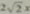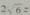### 九年级数学上册第4章《一元二次方程》4.5 一元二次方程根的判别式（复习与巩固）

• 同步课程
• 本节重点
• 主讲老师

4.5 一元二次方程根的判别式

1.不解方程，判断下列方程根的情况：
（1）2x²+x+1/4=0；（2）4t（t-1）=3；
（3）x²+2=（4）3z²-=-2
2.关于x的方程x²+ax-1=0有没有实根？如果有，两个实根是否相等？
3.已知关于x的方程mx²+mx+5=m有两个相等的实根，求m的值.
mx²+mx+5=m
mx²+mx+5-m=0
△=0
m1=0 m2=4且m≠0
m²-4m（5-m）=0
m²-20m+4m²=0
5m²-20m=0
5m（m-4）=0
4.当k为何值时，关于y的方程（k-1）y²-2ky+k=3.
（1）有两个不相等的实根；
（2）有两个相等的实根；
（3）没有实根.
5.已知关于x的方程kx²-4kx+k-5=0有两个相等的实根，解这个方程.
b²-4ac=（-4k）²-4k（k-5）=0
k1=0（舍去）k2=-5/3
-5/3x²+20/3x-20/3=0
5x²-20x+20=0
x²-4x+4=0
6.已知a，b，c是△ABC的三条边的长.求证：关于x的方程
cx²-（a+b）x-c/4=0

∵△=[-(a+b)]²-4c×c/4
=a²+2ab+b²-c²
=（a+b）²-c²
=（a+b+c）（a+b-c）
∵a，b，c为△ABC边长
∴△＞0